#Function Repository Resource:

# BranchPairs

Compute branch pairs for a given multiway system

Contributed by: Jonathan Gorard
 ResourceFunction["BranchPairs"][rules,init,n] generates a list of branch pairs for the multiway system with the specified rules after n steps, starting with initial conditions init. ResourceFunction["BranchPairs"][rules→sel,init,n] uses the function sel to select which of the events obtained at each step to include in the evolution.

## Details and Options

Rules can be specified in the following ways:
 {"lhs1"->"rhs1",…} string substitution system {{l11,l12,…}->{r11,r12,..},…} list substitution system CellularAutomaton[rules] cellular automaton system "type"→rules system of the specified type
Supported rule types include:
 "StringSubstitutionSystem" rules given as replacements on strings "ListSubstitutionSystem" rules given as replacements on lists "CellularAutomaton" rules given as a list of CellularAutomaton rule specifications "WolframModel" rules given as replacements on hypergraphs
When rules are specified by an explicit association, the following elements can be included:
 "StateEvolutionFunction" gives the list of successors to a given state "StateEquivalenceFunction" determines whether two states should be considered equivalent "StateEventFunction" gives the list of events applicable to a given state "EventApplicationFunction" applies an event to a given state "EventDecompositionFunction" decomposes an event into creator and destroyer events for individual elements "SystemType" gives a system type name "EventSelectionFunction" determines which events should be applied to a given state
The event selection function sel in ResourceFunction["BranchPairs"][rulessel,] can have the following special forms:
 "Sequential" applies the first possible replacement (sequential substitution system) "Random" applies a random replacement {"Random",n} applies n randomly chosen replacements "MaxScan" applies the maximal set of spatially-separated replacements (strings only)
The initial condition for ResourceFunction["BranchPairs"] is a list of states appropriate for the type of system used.
ResourceFunction["BranchPairs"][rules,"string",] is interpreted as ResourceFunction["BranchPairs"][rules,{"string"},].
ResourceFunction["BranchPairs"] accepts both individual rules and lists of rules, and likewise for initial conditions.
Options for ResourceFunction["BranchPairs"] include:
 "IncludeStepNumber" whether to label states and events with their respective step numbers "IncludeStateID" whether to label states and events with unique IDs "GivePredecessors" whether to label branch pairs with their predecessor state
In the theory of abstract rewrite systems, a critical pair (branch pair) is a pair of expressions that can be derived from a common expression by two different applications of a rewrite rule (i.e. either the same rule applied in two different places, or two different rules).
More generally, a branch pair indicates where a multiway evolution bifurcates.

## Examples

### Basic Examples (5)

Generate the list of all branch pairs (i.e. critical pairs) for two string substitution systems:

 In:=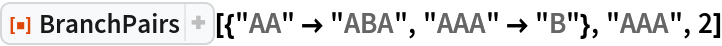Out=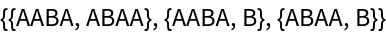In:=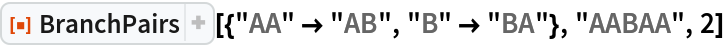Out=Show common predecessor states:

 In:=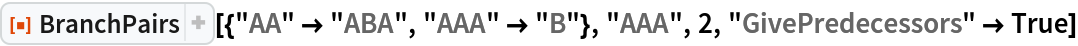Out=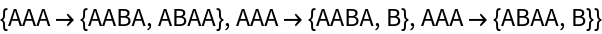Different event selection functions can lead to different lists of branch pairs:

 In:=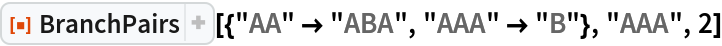Out=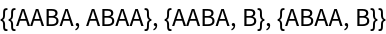In:=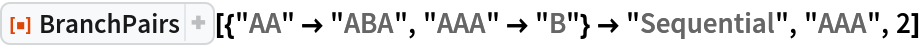Out=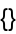BranchPairs can handle Wolfram Models and other system types:

 In:=Out=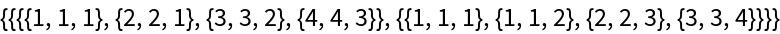In:=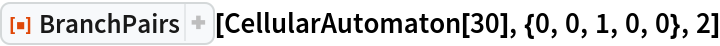Out=In:=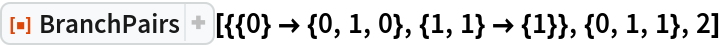Out=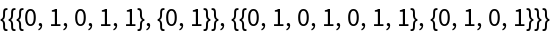Preventing identical states from being merged, by including step numbers and/or state IDs, can change branch pair behavior:

 In:=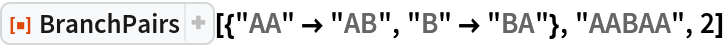Out=In:=Out=### Scope (10)

#### System Types (5)

BranchPairs supports both string and list substitution systems:

 In:=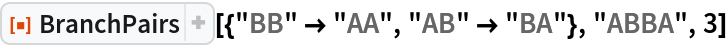Out=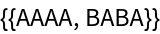In:=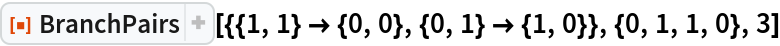Out=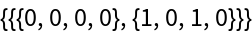Lists can contain arbitrary symbolic elements:

 In:=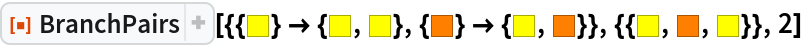Out=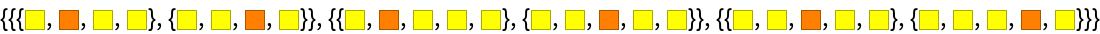Give an explicit substitution system rule:

 In:=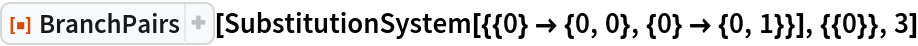Out=An alternative method of specifying that a substitution system should be used:

 In:=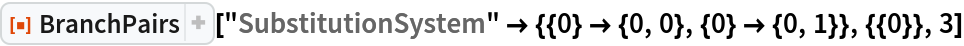Out=BranchPairs also supports multiway generalizations of cellular automata:

 In:=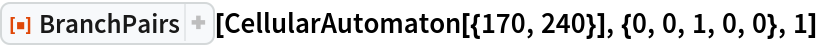Out=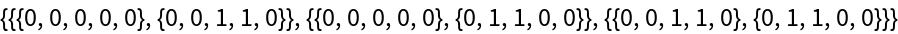Generate all branch pairs from left- and right-shift cellular automaton rules after 3 steps:

 In:=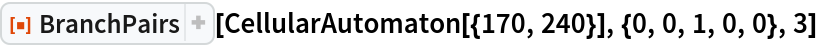Out=Determine branch pairs of the rule 30 cellular automaton:

 In:=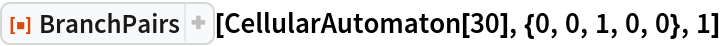Out=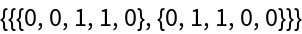BranchPairs also supports multiway generalizations of Wolfram Models:

 In:=Out=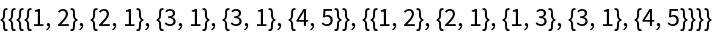In:=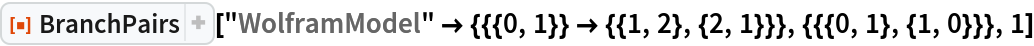Out=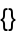Construct a multiway evolution by explicitly specifying an association:

 In:=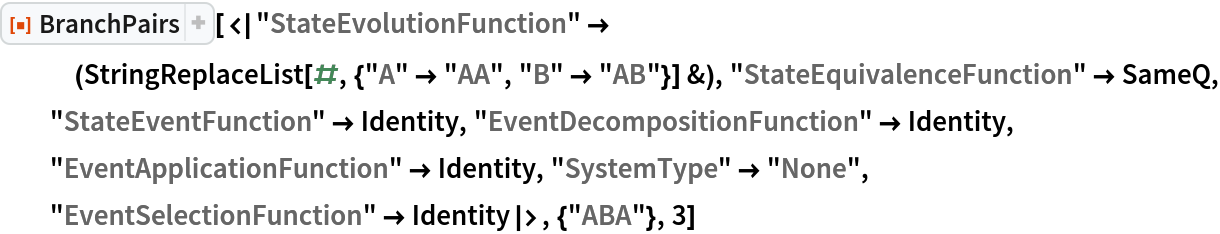Out=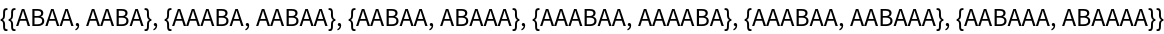#### Rules and Initial Conditions (2)

BranchPairs accepts both individual rules and lists of rules:

 In:=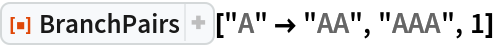Out=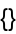In:=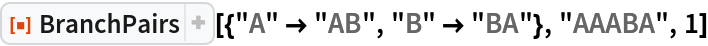Out=Likewise for initial conditions:

 In:=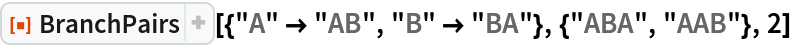Out=#### Event Selection Functions (3)

Apply only the first possible event at each step:

 In:=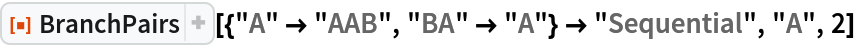Out=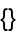Apply the first and last possible events at each step:

 In:=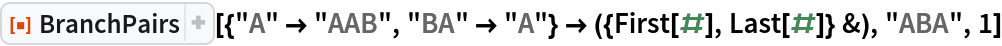Out=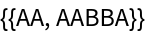Use a greedy-style algorithm to apply the maximal set of non-conflicting events at each step (strings only):

 In:=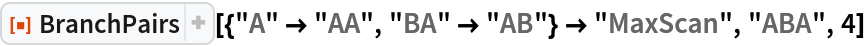Out=### Options (5)

Explicitly specify the type of rule:

 In:=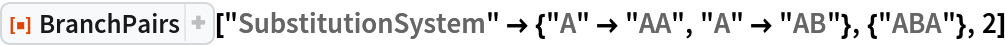Out=In:=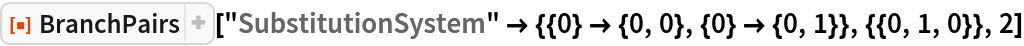Out=#### Step Numbers and State IDs (3)

By default, equivalent states are merged across all time steps:

 In:=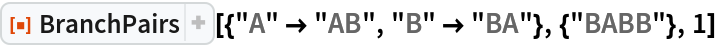Out=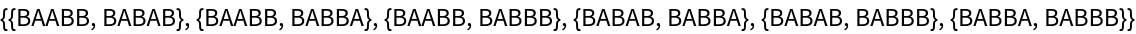Merging of equivalent states across different time steps can be prevented by including step numbers:

 In:=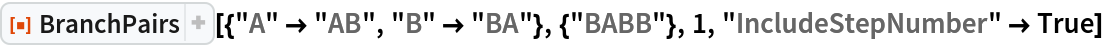Out=Merging of equivalent states at the same time step can be prevented by also including state IDs:

 In:=Out=#### Predecessor States (2)

By default, BranchPairs returns only a list of branch pairs:

 In:=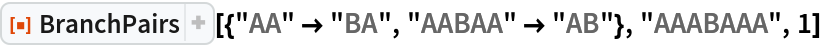Out=Common predecessor states can be shown using "GivePredecessors":

 In:=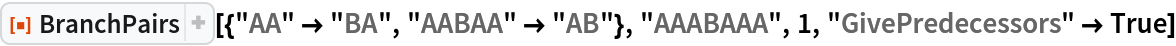Out=